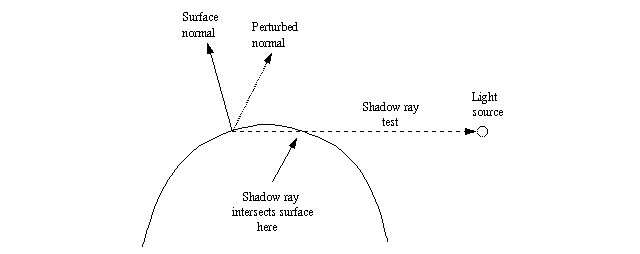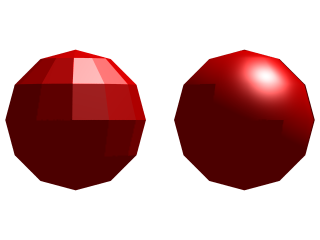POV-Ray : Documentation : 1.4.8.2 What causes the problem?POV-Ray 3.6 Documentation Online View#### 1.4.8.2 What causes the problem?

Let's start with the sphere with the perturbed normal, since it is easier to explain.This image shows graphically what happens.

The problem happens in the "dark side" of the object, that is, the side which does not "see" the light source.

Although the surface normal points away from the light source (ie. its angle is >90 degrees from the light source), the perturbed normal points towards it (ie. its angle is <90 degrees) and thus, according to the normal vector, the light source should illuminate the point in question.

However, when doing the shadow-ray test, POV-Ray sees that the test ray intersects with a surface (in this case the surface of the same sphere, but at the "other side"). Thus it decides that the surface in question is shadowing the current point and thus the light source does not illuminate it.

This is what causes the straight shadow exactly where the (non-perturbed) surface normal is exactly at 90 degrees from the light source.

The problem with the mesh of smooth triangles is a bit more difficult, although very similar (and caused by the exact same problem).This image shows graphically what happens.

Although there is no explicit normal perturbation, the fact that the surface is a mesh of smooth triangles means that there is an implicit normal perturbation.

In order to get a smooth appearance, each vertex has a normal vector and the normal vector at any point in the surface of the triangle is calculated by interpolating the normal vectors of the vertices.

Here the problem happens when the shadow line should pass across a triangle and the unperturbed normal vector of that triangle points away from the light source. As seen in the figure, a triangle that is closer to the light source will shadow the point in the current triangle (it is not necessarily the adjacent triangle, but if the mesh is closed, some triangle will surely shadow the point in question).

This means that this unfortunate triangle will be completely shadowed, thus causing a triangular artifact in the shadow line of the mesh.The image on the left shows more clearly why the shadow line of the smooth triangle mesh is like it appeared in the first image of this page.

The object at the left is the same triangle mesh, but with flat triangles, and the object at the right is the same object as in the image at the beginning of this page.

Notice how the shadowed triangles of the flat mesh correspond exactly to the artifacts in the shadow line of the smooth mesh. The reason for this was explained in the figure above.